# Special Chapters of Stochastic Processes and Applications

Autors: Maria Tudor, Mariana Sibiceanu, Bogdan Iftimie, Iulian Mircea

Year of appearance: 2011

ISBN: 978-606-505-395-3

31,80 lei In stock: YES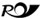Romanian Post delivery

The monograph Special Chapters of Stochastic Processes and Applications is concerned with stochastic processes which are of much interest  through  their applications in different economic fields, such as insurance and financial markets.

This book is divided in 6 chapters. In the first chapter are presented basic notions of Markov chains: the Markov property, the strong Markov property, classification of the states, limit theorems, finite Markov chains, examples. In chapter 2 is considered the filtering (estimation) problem of the real states of a system, based on the observed states. The discrete version of the Kalman-Bucy filtration algorithm  is also presented. A modern and rigorous mathematical tool used in financial modelling is represented by martingales. Chapter 3 is concerned with basic theory of martingales, such as the stopping theorem in discrete time, the discrete stochastic integral and the discrete stochastic exponential. In chapter 4 the fundamental concepts of financial market are analyzed, such as viable and complete financial markets.  There are characterized viable and complete markets in the case of  linear models associated to  financial markets. In chapter 5 we study Markov processes. Markov processes with most countable set of sets in continuous time are first considered, and as applications the Poisson process, the compound Poisson process and the birth and death processes are presented. The Brownian Motion is studied, as the most important stochastic process with arbitrary set of states in continuous time. Chapter 6 is devoted to applications of stochastic processes in insurance. There are presented the process of the number of claims, actuarial risk models of risk in discrete time and in continuous time.

This academic text assumes from the reader prerequisites of Probability Theory, which are usually taught in licence. A number of results which are frequently used are given at the end of the monograph (see Apendix A and Appendix B). It is addressed mostly to students in licence and master programs in Economics (such as Finance, Actuarial Theory, Cybernetics, Statistics). It is also recommended for students of the faculty of Mathematics, Ph.D students and specialists interested in applications of stochastic processes.

## Similar products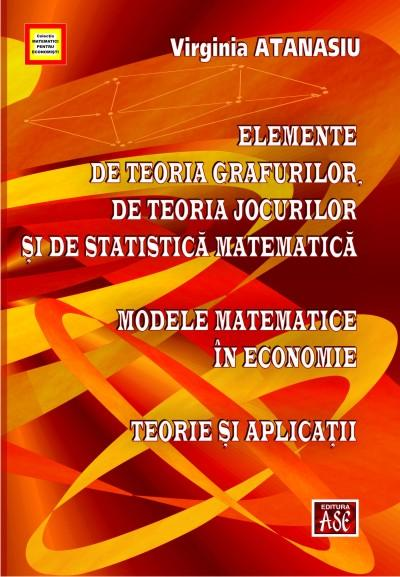### Elements of graph theory, game theory and mathematical statistics. Mathematical models in economics. Theory and Applications

53,00 lei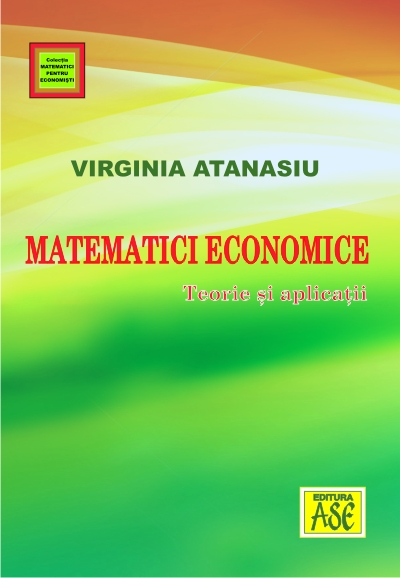### Matematici economice. Teorie si aplicatii

70,00 lei17,30 lei

## Others have bought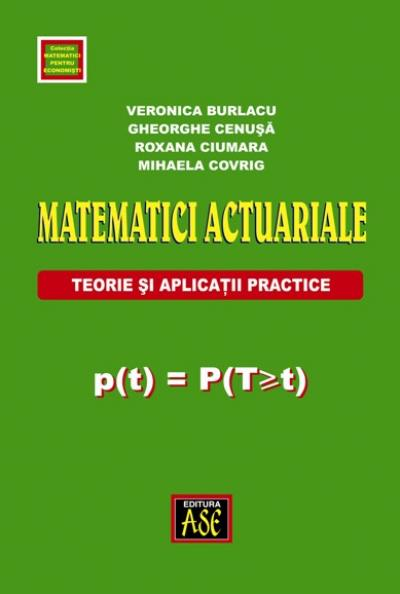### Actuarial Mathematics. Theory and practical applications

29,10 lei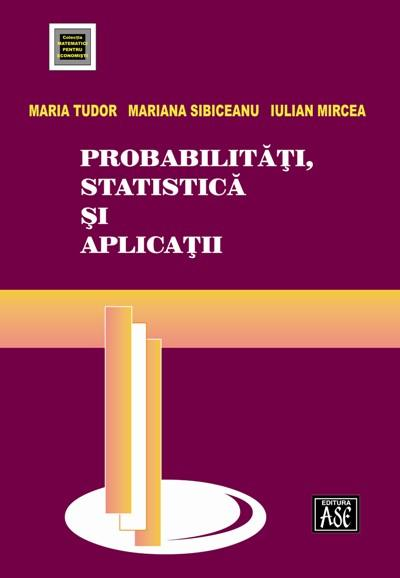### Probability, statistics and applications

35,80 lei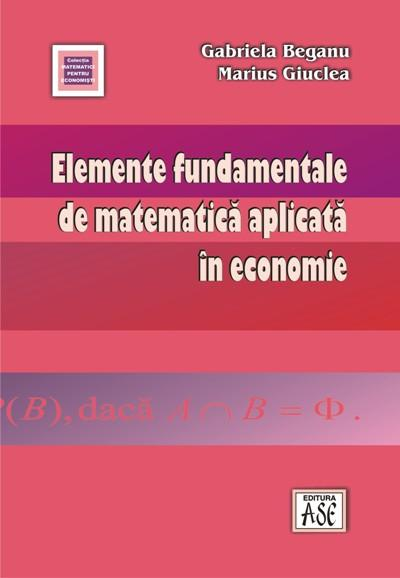### Fundamentals of applied mathematics in economics

20,70 lei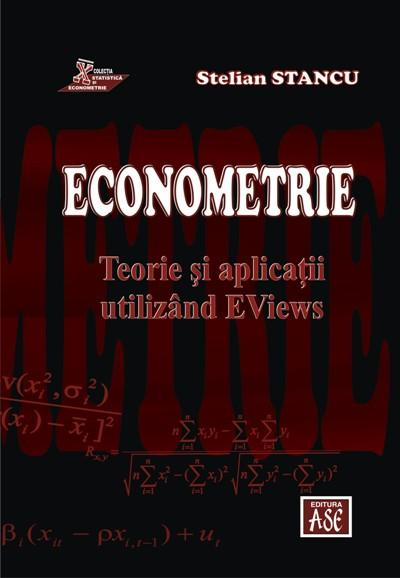38,50 lei